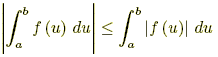# indefinite integral rules

Integral Rules

For the following, a, b, c, and C are constants; for definite integrals, these represent real number constants. The rules only apply when the integrals exist.

Indefinite integrals (These rules all apply to definite integrals as well)

1.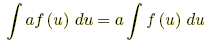2.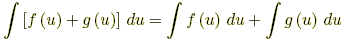3.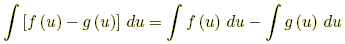4.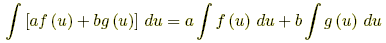5. Integration by parts: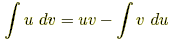Definite integrals

1.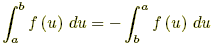2.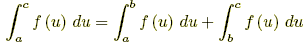3. If f(u) g(u) for all aub, then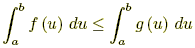4. If f(u) M for all aub, then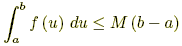5. If m f(u) for all aub, then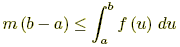6. If ab, then